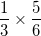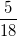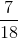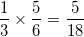# ISEE Lower Level Quantitative : Operations with fractions and whole numbers

## Example Questions

### Example Question #1691 : Ssat Middle Level Quantitative (Math)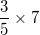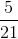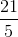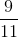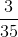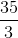Explanation:When we multiply a fraction by a whole number, we first want to make the whole number into a fraction. We do that by putting the whole number over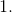Then we multiply like normal.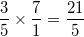### Example Question #1692 : Ssat Middle Level Quantitative (Math)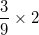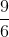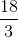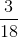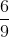Explanation:When we multiply a fraction by a whole number, we first want to make the whole number into a fraction. We do that by putting the whole number overThen we multiply like normal.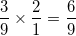### Example Question #1693 : Ssat Middle Level Quantitative (Math)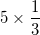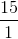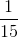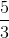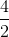Explanation:When we multiply a fraction by a whole number, we first want to make the whole number into a fraction. We do that by putting the whole number overThen we multiply like normal.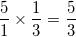### Example Question #251 : Fractions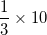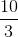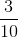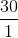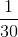Explanation:When we multiply a fraction by a whole number, we first want to make the whole number into a fraction. We do that by putting the whole number overThen we multiply like normal.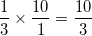### Example Question #1695 : Ssat Middle Level Quantitative (Math)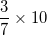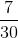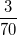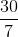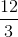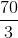Explanation:When we multiply a fraction by a whole number, we first want to make the whole number into a fraction. We do that by putting the whole number overThen we multiply like normal.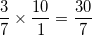### Example Question #252 : Fractions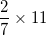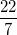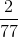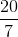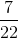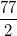Explanation:When we multiply a fraction by a whole number, we first want to make the whole number into a fraction. We do that by putting the whole number overThen we multiply like normal.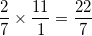### Example Question #21 : Operations With Fractions And Whole Numbers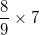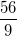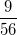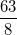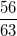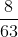Explanation:When we multiply a fraction by a whole number, we first want to make the whole number into a fraction. We do that by putting the whole number overThen we multiply like normal.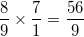### Example Question #614 : Fractions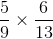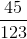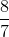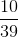Explanation:

When we multiply fractions, we multiply the numerator by the numerator and the denominator by the denominator.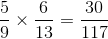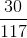can be reduced toby dividing both sides by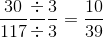### Example Question #1281 : Numbers And Operations

Solve: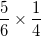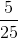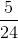Explanation:

When we multiply fractions, we multiply the numerator by the numerator and the denominator by the denominator.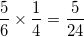### Example Question #31 : Multiply A Fraction Or Whole Number By A Fraction: Ccss.Math.Content.5.Nf.B.4

Solve: